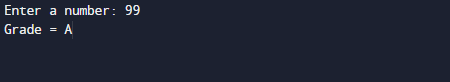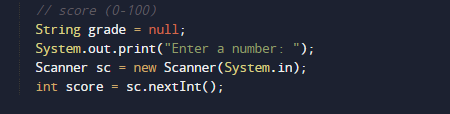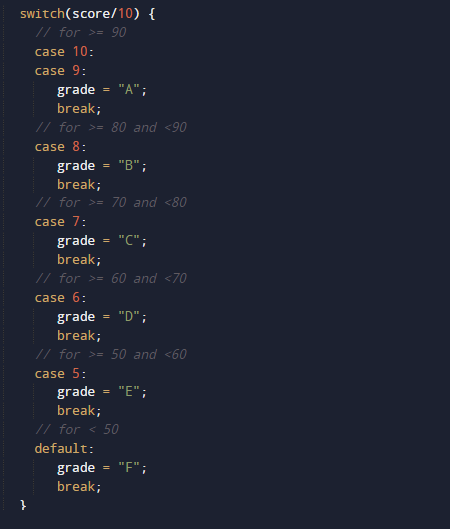# Java Program to Find Students Grades using Switch Case

In this tutorial you will learn about the Java Program to Find Students Grades using Switch Case and its application with practical example.

In this tutorial, we will learn to create a Java program to Find students Grade using Switch Case using Java programming.

## Prerequisites

Before starting with this tutorial we assume that you are best aware of the following Java programming topics:

• Java Operators.
• Basic Input and Output
• Class and Object.
• Basic Java programming.
• if-else statements.
• Switch case.
• Nested if-else Statements.
• Java Scanner class.

Here we are going to write a Java program to find out students grades using switch case statement. The Table shows the grading system.

## Java Program to Find Students Grades using Switch Case

In this our program we will create a program to find Student Grades using Switch Case. We would first declared and initialized the required variables. Next, we would prompt user to input value.Later in the program we will find Garde.

## OutputIn the above program, we have first declared and initialized a set variables required in the program.

• score =it will  holds value of score given by user.